•Gang Tao

# Real-time Machine Learning with Streaming SQL

Updated: Jun 23

SQL + Streaming + ML: Three great tastes that taste great together

Good engineering solves problems directly and elegantly. Having spent a lot of time recently with streaming data, I’ve seen how many data engineers, data scientists, and developers struggle to bring together the diverse skill sets and tooling required to apply ML to real-time data. It is a tricky problem, for sure. In today’s blog, I’ll show how you can use existing tools to run common ML use cases on streaming data.I love making ice cream sundaes with my kids. Nothing better than putting together different flavors of ice cream, sprinkles, and chocolate sauce to make my kids smile. My approach to building real-time ML is similar: I fuse three great tools, which wind up working even better when put together. What are the key components? First, SQL. It’s one of the world's most commonly used languages, and is easy to use. Streaming processing offers you the ability to analyze real-time with ultra-low latency. Machine learning empowers you to make accurate predictions about your data. Putting SQL + Streaming + ML together enables you to develop predictive applications on real-time data easily.

## Streaming SQL for Machine Learning

In today’s article, I’ll talk about how to use streaming SQL to run machine learning algorithms to do real-time prediction, anomaly detection and recommendations.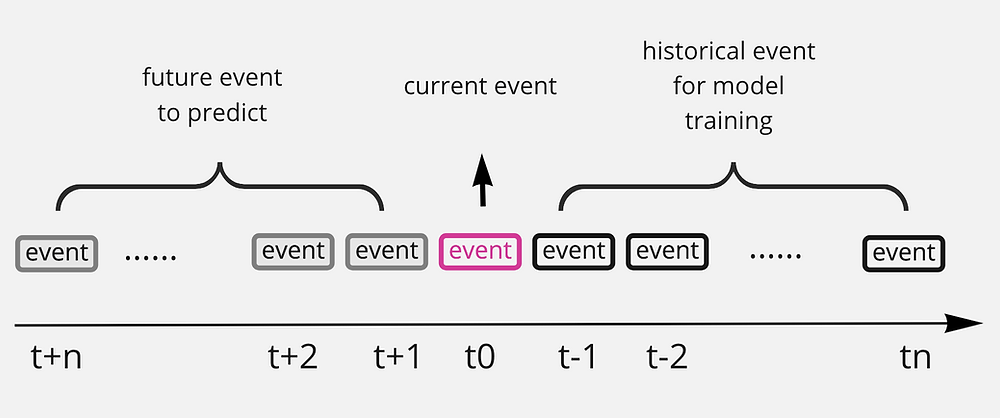In the streaming case, all the events are processed in real-time (see diagram above). Historical events will be used to build the model to predict future or current events.

In traditional ML applications, the model is training with a volume training dataset in a batch model. Usually it takes a long time to train a good model. In real-time scenarios, we leverage newly generated data to build and iterate on the model continuously. This allows practitioners to emphasize recent data - so-called “hot data” - more efficiently.

In the following sample, we train the model in real-time. Leveraging streaming SQL query, events are pushed to the user with every incoming event. A new model is trained using relative historical data to this incoming event; a lag function is used to get the past event sequence which is relatively new and abandon all the old data. To make things simple, we use 5 past events to explain this. So t0 is the current event, a model is trained using [t-1, t-2, t-3, t-4, t-5] , so this model is updated every time a new event is coming. This model can be used to predict the current event or the future event.

To help you familiarize you with the tools, all the following cases are based on sample car demo scenario which you can try on Timeplus’s playground. Timeplus’s car demo is a simulated scenario where a company is running a car sharing business and collecting real-time car sensor data for analysis purposes. The main stream that contains the car sensor data is called `car_live_data` and our following demos are all based on this stream. This stream contains following information:If you run following query in the playground, `select * from car_live_data` , you will get:The query result contains all the events from `car_live_data` and is pushed to the query client in real-time.

## Case 1: Real-time Prediction with Linear RegressionImage from Machine Learning Models Explained to a Five-year-old (Dibble)

The first case is to predict the amount of gas left in a specific car using a linear regression model.

Linear regression is a linear approach for modeling the relationship between a scalar response and one or more explanatory variables.

Linear regression is one of the most well known machine-learning algorithms. It assumes the predicted target has a linear relationship with the features in the data. In our demo, we assume the gas left in a car is correlated with time, so we can use the past gas usage to predict the gas left in a future time.

A regression line is simply a line:that is able to compute an output variable y for an input variable x . A line can be described by two parameters, also called coefficients:

• the slope m

• the intercept b

The m and b are computed via two equations:Here and overline y and overline x are the averages for y and x.

WITH LinearModel AS ( SELECT gas_percent, to_int(time) AS t, [lag(t), lag(t, 2), lag(t, 3), lag(t, 4), lag(t, 5)] AS X, [lag(gas_percent), lag(gas_percent, 2), lag(gas_percent, 3), lag(gas_percent, 4), lag(gas_percent, 5)] AS Y, array_avg(X) AS avg_X, array_avg(Y) AS avg_Y, array_sum(array_map((x, y) -> ((x - avg_X) * (y - avg_Y)), X, Y)) / array_sum(array_map(x -> ((x - avg_X) * (x - avg_X)), X)) AS m, avg_Y - (m * avg_X) AS b FROM car_live_data WHERE cid = 'c00031' ) SELECT gas_percent, to_datetime(t), (t * m) + b AS predict FROM LinearModel

If you run above SQL in Timeplus, it will return the real-time predict result like this:You can see from the sample that the predicted value is very close to the real value.

Let me explain this how model is trained in this SQL:

• A linear regression model is built using stream `car_live_data` for one specific car with id `c00031` to use timestamp feature (x) to predict gas percentage (y)

• to_int(time) convert time field with datetime type to timestamp int type

• [lag(t), lag(t, 2), lag(t, 3), lag(t, 4), lag(t, 5)] is an array of the timestamp from past 5 events which is used as the feature (x) to train the linear regression model. The lag function return a past event fields according to the offset in the lag parameter

• [lag(gas_percent), lag(gas_percent, 2), lag(gas_percent, 3), lag(gas_percent, 4), lag(gas_percent, 5)] is an array of the gas percentage from past 5 events which is used as the predict target (y) to train the linear regression model

• array_avg is a function that calculates the average value from an array

• To calculate the slope m, we use two functions array_map and array_sum, you can find the reference doc from Timeplus’s website

The prediction is done in the same query SQL, where t is he current timestamp and (t*m + b) is the predicted gas.

Of course, drivers have survived for decades estimating the amount of gas in their vehicles without the use of machine learning: dashboards solve that problem just fine. We are just taking a simple example here for illustrative purposes; this methodology can be applied to any number of similar analyses requiring regression.

Replace the predict SQL part from the previous SQL with:

SELECT gas_percent, to_datetime(t) as now, array_map(x -> ((x * m) + b), [t + 600, t + 1200, t + 1800]) AS predicts FROM LinearModel

This will output the predicted gas percentage after 10/20/30 minutes in an array.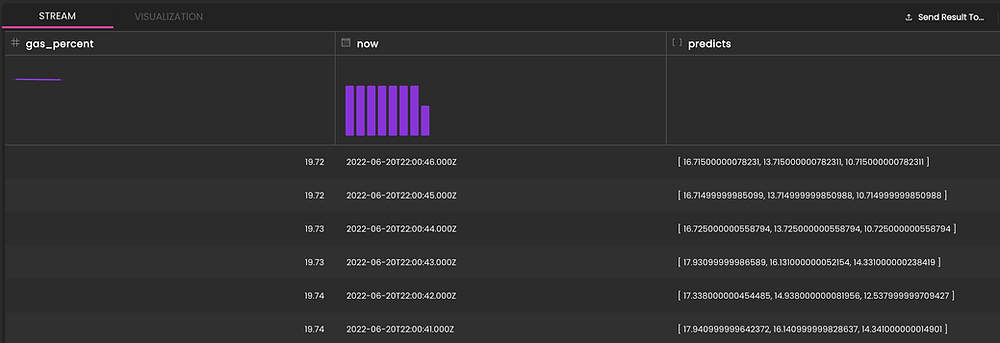Linear regression algorithm is a simple but powerful tool and you can easily apply it into scenarios like:

• Sales analysis such as pricing, performance, and risk parameters

• Consumer behavior analysis, profitability, and other business factors

• Trend analysis, making estimates, and forecasts

## Case 2: Real-time Anomaly Detection with Z-Score

Anomaly detection is one of the most common use cases in real-time machine-learning applications. An anomaly is a data point pr event that follows a different pattern or has different features than the majority of the data/events. An anomaly is usually connected with some business problems such as bank fraud, server down, equipment failure, to be simple, something is wrong and the user needs to take action.Some commonly used anomaly detection algorithms include: iso forest, one class svm, local outlier factor etc. In this example, I’ll show how to use z-score to detect anomalies in the car live events.

A Z-score is a numerical measurement that describes a value's relationship to the mean of a group of values. Z-score is measured in terms of standard deviations from the mean. If a Z-score is 0, it indicates that the data point's score is identical to the mean score. A Z-score of 1.0 would indicate a value that is one standard deviation from the mean. Z-scores may be positive or negative, with a positive value indicating the score is above the mean and a negative score indicating it is below the mean.

Z-score is defined as: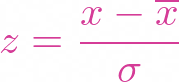where overline X the is the mean of the data value and σ is the standard deviation.Z-score could be positive or negative, a 0 Z-score means the value is equivalent to mean, a negative value means it is less than mean, and a positive value means it is bigger than the mean. The bigger the absolute value of the Z-score means it is far away from the mean value. The user must choose a threshold of the Z-score to decide whether an observation is an anomaly or not, in my sample, I use 2.5 a this thread hold but user can chose a different threshold here for the Z-score.

WITH Anomaly AS ( SELECT speed_kmh, [lag(speed_kmh), lag(speed_kmh, 2), lag(speed_kmh, 3), lag(speed_kmh, 4), lag(speed_kmh, 5)] AS X, array_avg(X) AS mean, sqrt(array_avg(array_map(x -> ((x - mean) * (x - mean)), X))) AS std, abs((speed_kmh - mean) / std) AS zscore FROM car_live_data WHERE cid = 'c00036' ) SELECT speed_kmh, zscore FROM Anomaly WHERE zscore > 2.5

If you run above SQL in Timeplus , you will get: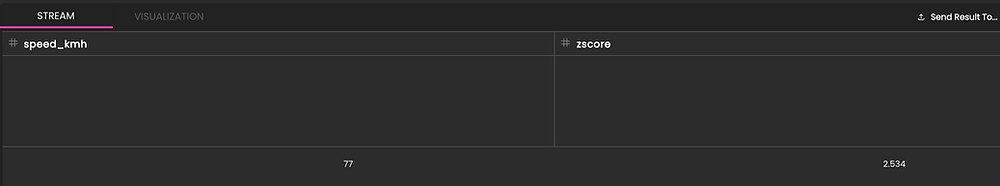Compared to the previous sample, this one is much easier to understand:

1. A Z-score based anomaly model is build using CTE clause after the WITH

2. speed_kmh is the feature used to build the model

3. If the Z-score of the current speed is bigger than 2.5, we believe it is an anomaly event , something might be wrong and action is needed.

4. Previous 5 events are retrieved by using [lag(speed_kmh), lag(speed_kmh, 2), lag(speed_kmh, 3), lag(speed_kmh, 4), lag(speed_kmh, 5)]

Beside array_map and array_avg which we have talked about, sqrt and abs are the two math functions used to calculate the square root and absolute value.

Z-score is a statistical analysis method. Z-Score-based anomaly detection is fast, easy to explain and easy to apply. In this example, 5 data points might be too small for a real case; more data points are required to build meaningful models. Z-Score-based anomaly detection is good for time-series data and monitoring use cases where you want to quickly find the outliers within massive sets of fast-changing streaming data.

## Case 3: Real-time Recommendations using KNN

Building an effective real-time recommendation system is the requirement of many businesses today.

There are two main strategies to do recommendations:

1. Collaborative filtering In this strategy, if user A and user B are similar, the system will recommend user A with what user B likes (buy, read)

2. Content-Based Filtering In this strategy, if the user used to like something such a A, the system will recommend the user with something similar to A

For whatever the strategy the system is using, the core problem to solve is how to find similarities between users/merchants. This is where the KNN is useful.Image from Machine Learning Models Explained to a Five-year-old (Dibble)

K Nearest Neighbors is a classification algorithm that can be used to group similar data together. As a core function of building a recommendation system, I will show how to run a KNN algorithm with Timeplus’s streaming SQL. In the following example, we use KNN algorithm to calculate the closest car based on the past car live event.

WITH KNN AS ( SELECT latitude, longitude, speed_kmh, cid, [lag(latitude), lag(latitude, 2), lag(latitude, 3), lag(latitude, 4), lag(latitude, 5)] AS X1, [lag(longitude), lag(longitude, 2), lag(longitude, 3), lag(longitude, 4), lag(longitude, 5)] AS X2, [lag(cid), lag(cid, 2), lag(cid, 3), lag(cid, 4), lag(cid, 5)] AS Y, array_map((x, y) -> sqrt(((latitude - x) * (latitude - x)) + ((longitude - y) * (longitude - y))), X1, X2) AS Distance, array_sort(x -> (x.1), array_zip(Distance, Y)) AS NearestNeighbors, array_map(x -> (x.2), NearestNeighbors) AS knn, array_distinct(knn) AS elements, array_map(x -> array_count(y -> (y = x), knn), elements) AS count, array_reverse(array_sort(x -> (x.2), array_zip(elements, count))) AS voting FROM car_live_data ) SELECT cid, voting FROM KNN

Run above SQL in Timeplus’s playground, you will get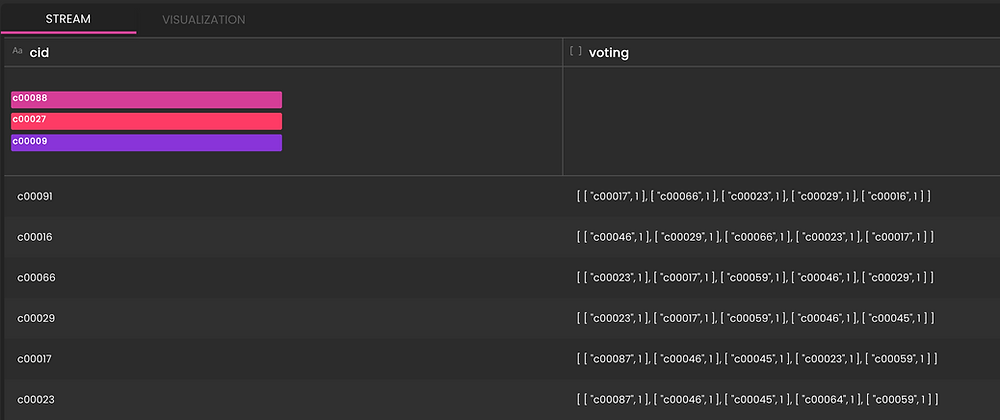The first part of the KNN algorithm is to get the distance between the target data point from all the training data points.

The CTE clause is used to calculate the distance between the current car and the car from the previous 5 events. And then find the most frequent occurrence of these cars which is the voting part of the KNN algorithm.The distance array is calculated using longitude and latitude by the above formula.

This array_sort(x-> x.1, array_zip(Distance, Y)) as NearestNeighbors is used to calculate the closest car by ordering with the distance. array_zip function will combine a calculated distance array and the cid array Y. array_sort will order the array using the first element in the zipped array which is the distance. (Note, in timeplus, the array/tuple index start with 1, not 0, this is commonly used in SQL)

Here is a sample output of the NearestNeibors , which is ordered by the distance.

```[
[136.4279277179886,"c00086"]
[187.51456263328194,"c00053"]
[197.3117767754148,"c00068"]
[232.72891998042473,"c00021"]
[244.41345260999915,"c00066"]
]```

array_map(x -> (x.2), NearestNeighbors) AS knn, will get only those cid fields from NearestNeighbours for next step processing.

```[
"C00086",
"C00053",
"C00068",
"C00021",
"C00066"
]```

array_distinct(knn) AS elements, will return the distinct elements from the array knn, we will use this elements array to check the occurrence of each element. In our case, as there are thousands of cars reporting events, these previous 5 events are most likely reported from different cars, so the distinct ids are the same as the original one. But in the real KNN use cases, usually there might exist repeated elements.

The second part of KNN algorithm is voting, which finds the most frequent occurrence from all the nearest neighbors.

array_map(x -> array_count(y -> (y = x), knn), elements) AS count this line of SQL calculate the occurrence of each car from NearestNeighbours using array_map and array_count.

Last, with array_reverse(array_sort(x -> (x.2), array_zip(elements, count))) the voting result is ordered so we know which car has the most frequency of occurrence.

Although this sample is not exactly a recommendation use case, it is a complete streaming SQL implementation of KNN algorithm that can be used to build recommendations with your streaming data.

KNN algorithm is a well known classification algorithm which can be used when you need to search for similarities. The recommendation system is one of the typical applications using KNN, but it can also be used in some other applications such as user segment analysis, text similarity analysis etc.

## Summary

As mentioned, I’ve been through the pain of trying to build ML applications for real-time data. That inspired me to build a tool that would enable ML practitioners to deploy ML applications more quickly and cost effectively than what I had struggled with! I think our team at Timeplus has succeeded in this task. Specifically, in this blog we’ve shown how our Streaming SQL can support three typical real-time-data machine learning use cases. Linear Regression for Prediction, ZScore for Anomaly Detection, and KNN for recommendation. These algorithms are easy to integrate and express as pure SQL.

Those features are the key to support real-time machine learning algorithm to be implemented:

• Event base streaming query Query event are pushed per event based, so the user can train the mode and do the predication in real-time upon arrival of each event

• Past event sequence retrieving Lag functions support the user to retrieve historical events used for model training.

• Easy to use and powerful math and array function Math functions like sqrt, abs and Array functions like array_map, array_sum enable the user to implement different equations easily with streaming data

The technique shown in this blog can be used in a lot of scenarios where you don't need to recursively train a complex model, and you dont need keep state for the trained models, such as real-time monitoring, anomaly detections, recommendations, regression analysis with time series data. While it does not apply to the case where a complex model needs to be trained recursively because of the lack of interaction in descriptive SQL language. So if you need to do something like a deep learning application such as NLP or image recognition, this technique does not apply. To support such use cases, User Defined function (UDF) will be helpful. With UDF, the user can keep trained ML model and state in the backend service to support more sophisticated ML models. We can talk about how to use UDF in Timeplus later on. Here is a sample of using UDF to do the prediction:

SELECT gas_percent, predict([total_km,speed_kmh],gas_percent, 'model') as gas_percent FROM car_live_data

The predict function here is a user defined function, you can see, using a user defined function can greatly simplify the SQL and support stateful ML training. While the user has to maintain a backend function to support this which could be a AWS lambda function or a RESTful Web Service.

The techniques shown in this blog can be used in a lot of scenarios where you don't need to recursively train a complex model, and you don't need to keep state for the trained models, such as real-time monitoring, anomaly detections, recommendations, regression analysis with time series data. It does not apply to cases where a complex model needs to be trained recursively because of the lack of interaction in descriptive SQL language. So if you need to do something like a deep learning application such as NLP or image recognition, this technique does not apply. To support such use cases, User Defined function (UDF) will be helpful. With UDF, the user can keep a trained ML model and state in the backend service to support a more sophisticated ML model. We can talk about how to use UDF in Timeplus later on.

All the samples in this article can be run in Timeplus’s public playground without registration. If you are interested in building real-time applications, you can learn more about how Timeplus can support you at our website.

48 views